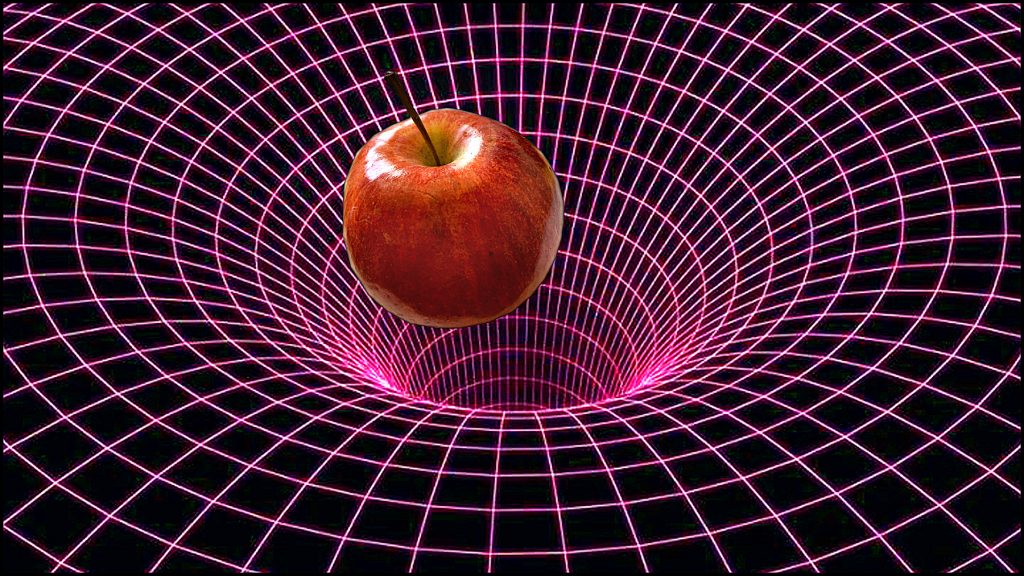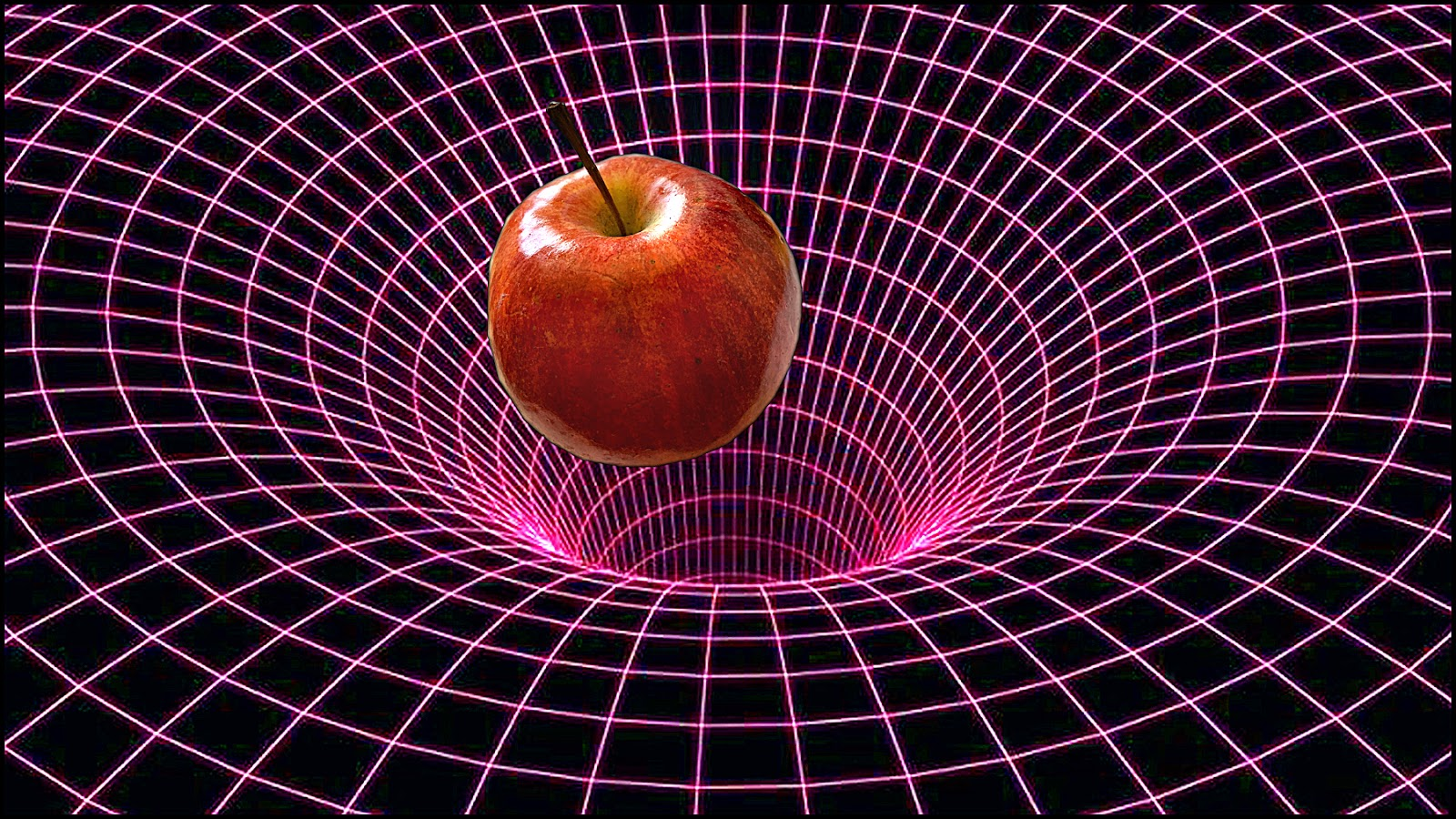# Difference of Energy, Force, Work. Power, Gravity and Mass

All types of matter contain energy and mass so what makes them different. We can do work using a force and measure the power of that work. But energy cannot be created or destroyed and that makes things difficult to understand. Energy, work and force are related to each other in physics so let’s define what they are and how they exist.Gravity is a force measured in Newtons.

Einstein’s famous equation E=mc^2 shows the equivalence between energy and mass. You can convert energy into things or convert mass into energy but in that process you can’t destroy or create energy in any closed system. If that system is the Universe the law still applies.

Energy is the potential or ability to do work. It is measured in Joules and 1 Joule is the amount of work done using a force of 1 newton. One newton is the force needed  to move 1 kg mass 1 metre per second per second.

Energy doesn’t exist alone, it needs something to work with. Energy and mass are related, neither one exists separately from the other. You can’t create energy without using mass and you can’t create mass without using energy in a closed system, such as the universe.

## Examples of Different Energies

Chemical energy is released when matter such as gasoline undergoes a chemical change during combustion to power your engine and move your car.

Electrical energy is measured in kilowatts by the movement of electrons that provide power to motors and light bulbs. The work done in a Kilowatt of power is equal to 3,600 kilo-Joules.

Mechanical energy is the ability to work and move an object using mechanical force. Contact force is like from a spring inside your pen and non contact force is like a hammer hitting a nail or wind moving a fan.

Nuclear energy is fusion energy like inside the sun and fission energy inside atomic power plants.

Gravitational energy is using the mass of an object falling in a gravity field. A common example is a hydro dam creating electrical energy. On Earth the force of gravity is 9.8 metres per second per second which is 9.8 newtons. One newton is the force needed to move a 1 kg mass 1 metre per second per second.

Thermal energy is used when a hot substance such as water is used as a force to provide work as in a hot spring electric generating station.

Solar energy is using the light from the sun to produce electricity. Plants and trees use sunlight that is converted into stored energy.

Magnetic energy is produced by electrical current of magnetic material containing iron.

Motion energy of wind or water can be used to move a generator to produce electricity.

Maybe you can think of other forms of energy. How about sexual energy ha ha ha…

## The Energy of Work

Work, energy and force are all related to the amount of mass of matter. Energy is the potential to do work, while work is a measure of the force used in the energy. The amount of energy is equal to the amount of work done.

This can be confusing because they are measured at different times using the same matter and mass of the object.

Work is measured in Joules since work is the product of the energy to move a mass. The amount of work that is done is calculated by the force (Newtons) times the distance to move a mass.

One newton is the force needed to move a 1 kg mass 1 metre per second per second. When work is done energy is transferred from one place or form into another place or form.

Gravity is measured in Newtons and on Earth as 9.8 newtons. Also we use the term 9.8 metres per second per second and the mass of an object doesn’t matter. But the work done depends on the mass.

The work using gravity is measured as force. Force equals mass times acceleration. Again we see that work, force and energy are all the same thing measured in different times and ways.

## The Power of Work

The power of work is measured by the number of joules per second or watts per second. Power equals work in joules measured during the time of one second. The unit for power is the watt, which equals 1 joule of work per second.

We usually convert the amount into horsepower. One horsepower is equal to 550 foot-pounds per second which is 745.7 watts.

I hope you can understand what gravity, mass, energy, force, power and work mean. They are all related by energy and mass to do work.

Remember, matter never minds and mind is never matter. Energy is paramount.

If you resonate with this new information I want you to be on my tribe of followers. I would like to encourage your dreams and confirm your thoughts that science of light will show us the Ultimate Truth.

I care about you and hope you will join this journey into understanding science and light.

Your influence is greater than you can imagine!

Join my list of subscribers and get my latest post in your email.

Thanks for being here. As always, be well. https://lovinthings.com

### About the Author Erik Lovin

Erik has a BSc degree and is a retired professional photographer who is now a published Author of many books. His passion is understanding how life and the universe works. He is currently blogging about the science of the Big Bang and the science of cosmology. Erik is helping his tribe with questions about the universe. His goal is to help find a theory of everything (TOE). In order to do that, he is trying to prove light has mass and that the fabric of spacetime is a false theory. We are welcoming questions and answers that you might have about the universe.